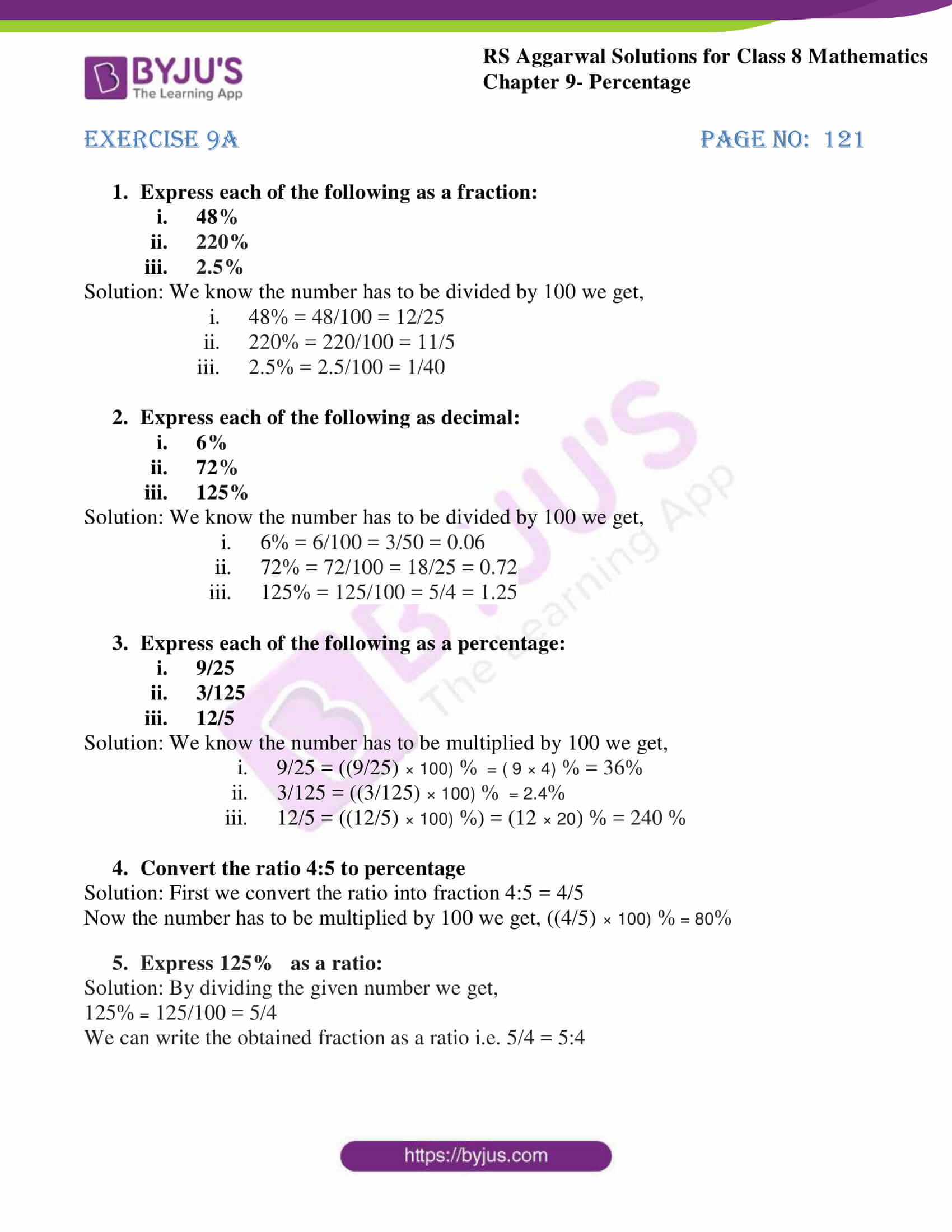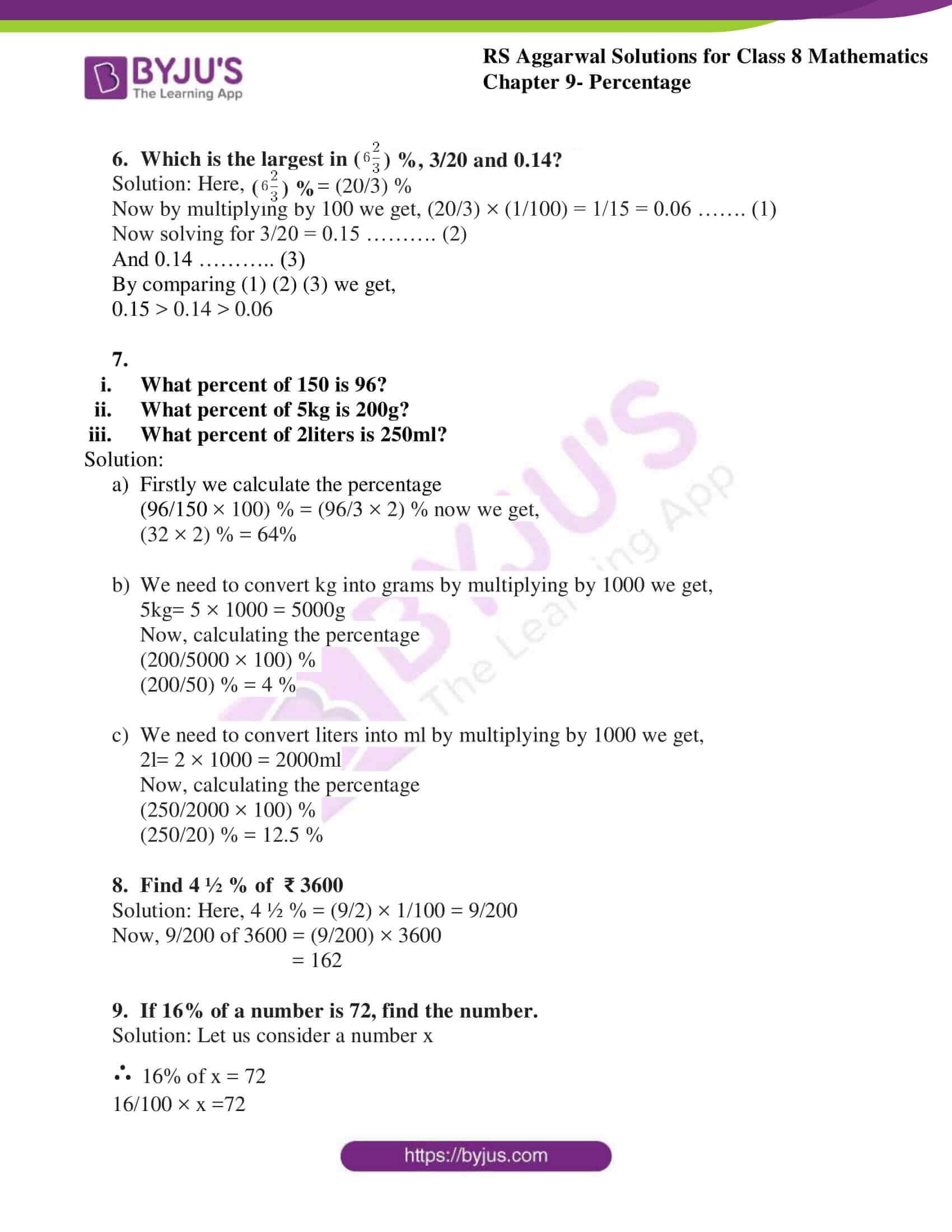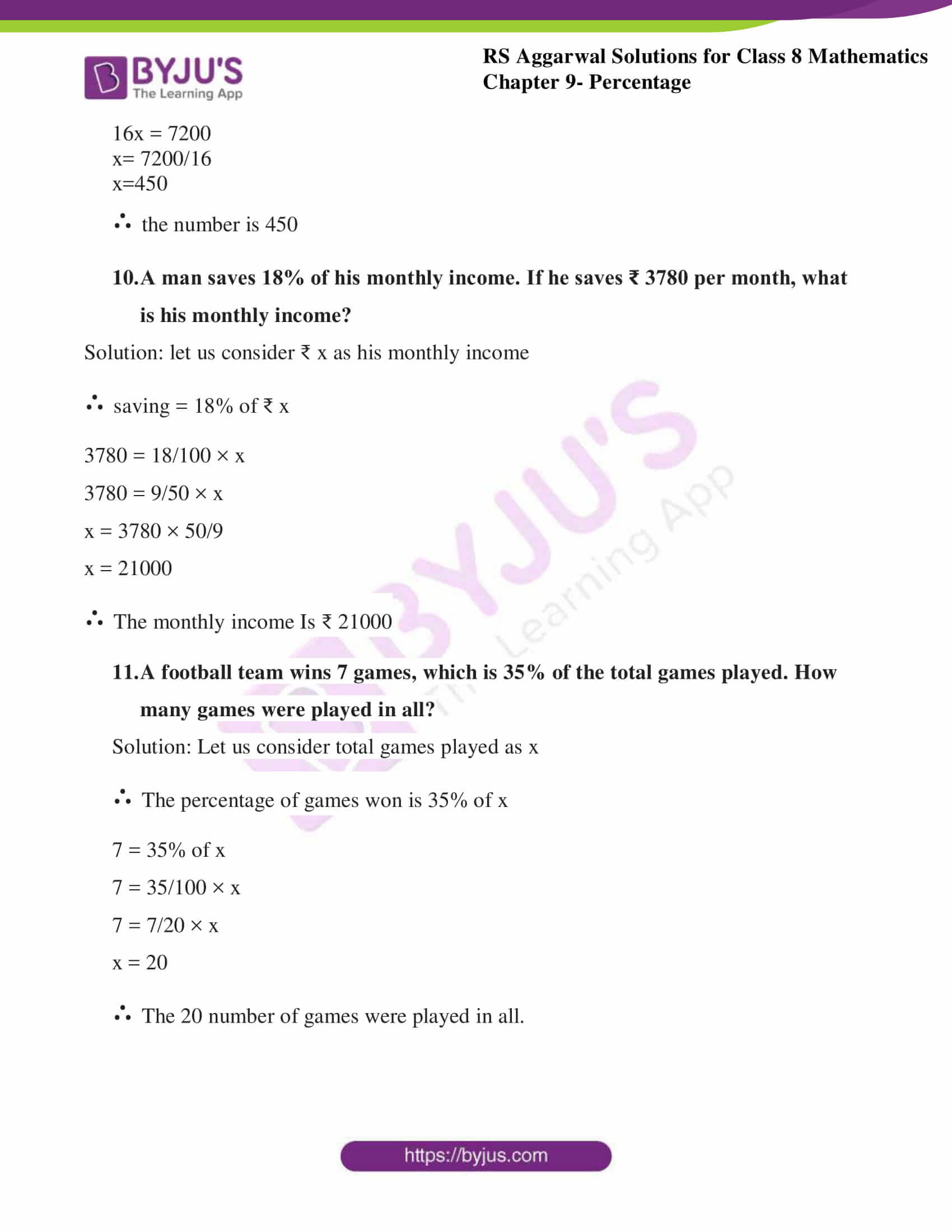# RS Aggarwal Solutions for Class 8 Maths Chapter 9 - Percentage Exercise 9A

From the links provided below, Students can refer and download RS Aggarwal Solutions for Class 8 Maths Chapter 9- Exercise 9A, Percentage. Our experts have solved the RS Aggarwal Solutions to ensure that the students are thorough with their basic concepts by practicing the solutions.

In Exercise 9A of RS Aggarwal Class 8 Maths, we shall discuss what is percentage and problems based on it. A percentage is a portion of a whole expressed as a number between 0 and 100 rather than a fraction. All of something is 100 per cent, half of it is fifty per cent, none of something is zero per cent. Mathematically we can say, by a certain per cent we mean that many hundredths.

## Download PDF of RS Aggarwal Solutions for Class 8 Maths Chapter 9- Percentage Exercise 9A### Access Answers to RS Aggarwal Solutions for Class 8 Maths Chapter 9- Percentage Exercise 9A

1. Express each of the following as a fraction:

1. 48%
2. 220%
3. 2.5%

Solution: We know the number has to be divided by 100 we get,

1. 48% = 48/100 = 12/25
2. 220% = 220/100 = 11/5
3. 2.5% = 2.5/100 = 1/40

2. Express each of the following as decimal:

1. 6%
2. 72%
3. 125%

Solution: We know the number has to be divided by 100 we get,

1. 6% = 6/100 = 3/50 = 0.06
2. 72% = 72/100 = 18/25 = 0.72
3. 125% = 125/100 = 5/4 = 1.25

3. Express each of the following as a percentage:

1. 9/25
2. 3/125
3. 12/5

Solution: We know the number has to be multiplied by 100 we get,

1. 9/25 = ((9/25) × 100) % = ( 9 × 4) % = 36%
2. 3/125 = ((3/125) × 100) % = 2.4%
3. 12/5 = ((12/5) × 100) %) = (12 × 20) % = 240 %

4. Convert the ratio 4:5 to percentage

Solution: First we convert the ratio into fraction 4:5 = 4/5

Now the number has to be multiplied by 100 we get, ((4/5) × 100) % = 80%

5. Express 125% as a ratio:

Solution: By dividing the given number we get,

125% = 125/100 = 5/4

We can write the obtained fraction as a ratio i.e. 5/4 = 5:4

6. Which is the largest in ($6\frac{2}{3}$) %, 3/20 and 0.14?

Solution: Here, ($6\frac{2}{3}$) % = (20/3) %

Now by multiplying by 100 we get, (20/3) × (1/100) = 1/15 = 0.06 ……. (1)

Now solving for 3/20 = 0.15 ………. (2)

And 0.14 ……….. (3)

By comparing (1) (2) (3) we get,

0.15 > 0.14 > 0.06

7. find:

1. What percent of 150 is 96?
2. What percent of 5kg is 200g?
3. What percent of 2liters is 250ml?

Solution:

1. Firstly we calculate the percentage(96/150 × 100) % = (96/3 × 2) % now we get,(32 × 2) % = 64%
2. We need to convert kg into grams by multiplying by 1000 we get,5kg= 5 × 1000 = 5000gNow, calculating the percentage(200/5000 × 100) %

(200/50) % = 4 %

3. We need to convert liters into ml by multiplying by 1000 we get,2l= 2 × 1000 = 2000mlNow, calculating the percentage(250/2000 × 100) %

(250/20) % = 12.5 %

8. Find 4 ½ % of ₹ 3600

Solution: Here, 4 ½ % = (9/2) × 1/100 = 9/200

Now, 9/200 of 3600 = (9/200) × 3600

= 162

9. If 16% of a number is 72, find the number.

Solution: Let us consider a number x

∴ 16% of x = 72

16/100 × x =72

16x = 7200

x= 7200/16

x=450

∴ the number is 450

10. A man saves 18% of his monthly income. If he saves ₹ 3780 per month, what is his monthly income?

Solution: let us consider ₹ x as his monthly income

∴ saving = 18% of ₹ x

3780 = 18/100 × x

3780 = 9/50 × x

x = 3780 × 50/9

x = 21000

∴ The monthly income Is ₹ 21000

11. A football team wins 7 games, which is 35% of the total games played. How many games were played in all?

Solution: Let us consider total games played as x

∴ The percentage of games won is 35% of x

7 = 35% of x

7 = 35/100 × x

7 = 7/20 × x

x = 20

∴ The 20 number of games were played in all.

### Access other Exercise of RS Aggarwal Solutions for Class 8 Maths Chapter 9- Percentage

Exercise 9B Solutions 20 Questions

## RS Aggarwal Solutions for Class 8 Maths Chapter 9- Percentage Exercise 9A

Exercise 9A of RS Aggarwal Class 8, Percentage. This exercise mainly deals with the percentage conversion to fraction, ratio and vice-versa.

The RS Aggarwal Solutions step by step ensures that students to get to know the best approach to the individual questions. By referring to these solutions, students develop problem-solving abilities and can tackle any type of questions easily. Students are suggested to practice the problems regularly which will help them excel in their exams and increase their overall percentage. Practicing as many times as possible helps in time management skills and also boosts the confidence level to achieve high marks.